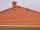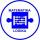# Seats

Seats in the sport hall are organized so that each subsequent row has five more seats. First has 10 seats. How many seats are:

a) in the eighth row
b) in the eighteenth row

Result

a =  45
b =  95

#### Solution:Leave us a comment of this math problem and its solution (i.e. if it is still somewhat unclear...):Be the first to comment!## Next similar math problems:

1. SequenceBetween numbers 1 and 53 insert n members of the arithmetic sequence that its sum is 702.
2. RootsDetermine the quadratic equation absolute coefficient q, that the equation has a real double root and the root x calculate: ?
3. Median and modusRadka made 50 throws with a dice. The table saw fit individual dice's wall frequency: Wall Number: 1 2 3 4 5 6 frequency: 8 7 5 11 6 13 Calculate the modus and median of the wall numbers that Radka fell.
4. RoofTiles are stacked in rows on the trapezoidal shaped roof. At the ridge is 15 tiles and each subsequent row has one more tile than in the previous row. How many tiled is covered roof if lowermost row has 37 tiles?
5. Sequence 3Write the first 5 members of an arithmetic sequence: a4=-35, a11=-105.
6. SequenceWrite the first 6 members of these sequence: a1 = 5 a2 = 7 an+2 = an+1 +2 an
7. AP - basicsDetermine first member and differentiate of the the following sequence: a3-a5=24 a4-2a5=61
8. Sequence 2Write the first 5 members of an arithmetic sequence a11=-14, d=-1
9. AS - sequenceWhat are the first ten members of the sequence if a11=22, d=2.
10. AP - simpleDetermine the first nine elements of sequence if a10 = -1 and d = 4
11. SequenceWrite the first 7 members of an arithmetic sequence: a1=-3, d=6.
12. Theorem proveWe want to prove the sentence: If the natural number n is divisible by six, then n is divisible by three. From what assumption we started?
13. AS sequenceIn an arithmetic sequence is given the difference d = -3 and a71 = 455. a) Determine the value of a62 b) Determine the sum of 71 members.
14. Third memberDetermine the third member of the AP if a4=93, d=7.5.
15. EquationEquation ? has one root x1 = 8. Determine the coefficient b and the second root x2.
16. Nineteenth memberFind the nineteenth member of the arithmetic sequence: a1=33 d=5 find a19
17. 75th percentile (quartille Q3)Find 75th percentile for 30,42,42,46,46,46,50,50,54